#### 11/23/20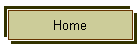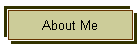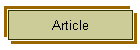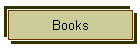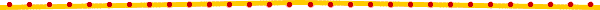Short introduction :

I am Dr.S.M.R.Hasheimi Moosavi.

I have discovered the formula of the prime numbers after 20 years of  research and injury. I solved the unsolvable problems related to them.Discovery  results :

The distinction of the prime numbers.

The generating formula of the prime numbers.

The definition of the prime numbers set by using the   generating function of prime numbers.

The generating formula of the Mersenne prime numbers.

The determining of the k-th prime number.

Solving Riemann zeta equation by using the determining of

the number of the prime numbers less than or equal arbitrary number (N) exactly.

Study of the guesses of Goldbuch and Hardy.

The proof of being infinity of the prime twin couples.Note: the results of this great discovery "the formula of generating

prime numbers discovery" has been sent to most of research centers

and universities of the world.What is a prime number?  A prime number is a positive integer that has exactly two positive integer factors, 1 and itself. For example, if we list the factors of 28, we have 1, 2, 4, 7, 14, and 28. That's six factors. If we list the factors of 29, we only have 1 and 29. That's 2. So we say that 29 is a prime number, but 28 isn't. Another way of saying this is that a prime number is a whole number that is not the product of two smaller numbers. Note that the definition of a prime number doesn't allow 1 to be a prime number: 1 only has one factor, namely 1. Prime numbers have exactly two factors, not "at most two" or anything like that. When a number has more than two factors it is called a composite number. Here are the first few prime numbers:   2, 3, 5, 7, 11, 13, 17, 19, 23, 29, 31, 37, 41, 43, 47, 53, 59, 61, 67, 71, 73, 79, 83, 89, 97, 101, 103, 107, 109, 113, 127, 131, 137, 139, 149, 151, 157, 163, 167, 173, 179, 181, 191, 193, 197, 199, etc.you can read about The Largest Known Primes on the Web#### What are Mersenne primes and why do we search for them?

A Mersenne prime is a prime of the form 2P-1. The first Mersenne primes are 3, 7, 31, 127, etc. There are only 41 known Mersenne primes.

GIMPS, the Great Internet Mersenne Prime Search, was formed in January 1996 to discover new world-record-size Mersenne primes. GIMPS harnesses the power of thousands of small computers like yours to search for these "needles in a haystack".

Most GIMPS members join the search for the thrill of possibly discovering a record-setting, rare, and historic new Mersenne prime. Of course, there are many other reasons.for more information about Mersenne primes , History ,Theorems and lists  click here .Why do people find these primes?

"Why?" we are often asked, "why would anyone want to find a prime that big?""  I often now answer with "did you ever collect anything?"" or "did you ever try to win a competition?"" Much of the answer for why we collect large primes is the same as why we might collect other rare items. Below I will present a more complete answer divided into several parts.

1. Tradition!

2. For the by-products of the quest

3. People collect rare and beautiful items

4. For the glory!

5. To test the hardware

6. To learn more about their distribution

This does not exhaust the list of reasons, for example some might be motivated by primary research or a need for publication. Many others just hate to see a good machine wasting cycles (sitting idle or running an inane screen saver).

Perhaps these arguments will not convince you.  If not, just recall that the eye may not see what the ear hears, but that does not reduce the value of sound.  There are always melodies beyond our grasp.

###1. Tradition!

Euclid may have been the first to define primality in his Elements approximately 300 BC. His goal was to characterize the even perfect numbers (numbers like 6 and 28 who are equal to the sum of their aliquot divisors: 6 = 1+2+3, 28=1+2+4+7+14). He realized that the even perfect numbers (no odd perfect numbers are known) are all closely related to the primes of the form 2p-1 for some prime p (now called Mersennes). So the quest for these jewels began near 300 BC.

Large primes (especially of this form) were then studied (in chronological order) by Cataldi, Descartes, Fermat, Mersenne, Frenicle, Leibniz, Euler, Landry, Lucas, Catalan, Sylvester, Cunningham, Pepin, Putnam and Lehmer (to name a few).  How can we resist joining such an illustrious group?

Much of elementary number theory was developed while deciding how to handle large numbers, how to characterize their factors and discover those which are prime. In short, the tradition of seeking large primes (especially the Mersennes) has been long and fruitful It is a tradition well worth continuing.

###2. For the by-products of the quest

Being the first to put a man on the moon had great political value for the United States of America, but what was perhaps of the most lasting value to the society was the by-products of the race.  By-products such as the new technologies and materials that were developed for the race that are now common everyday items, and the improvements to education's infrastructure that led many man and women into productive lives as scientists and engineers.

The same is true for the quest for record primes.  In the tradition section above I listed some of the giants who were in the search (such as Euclid, Euler and Fermat).  They left in their wake some of the greatest theorems of elementary number theory (such as Fermat''s little theorem and quadratic reciprocity).

More recently, the search has demanded new and faster ways of multiplying large integers.  In 1968 Strassen discovered how to multiply quickly using Fast Fourier Transforms. He and Schönhage refined and published the method in 1971. GIMPS now uses an improved version of their algorithm developed by the long time Mersenne searcher Richard Crandall .

The Mersenne search is also used by school teachers to involve their students in mathematical research, and perhaps to excite them into careers in science or engineering. And these are just a few of the by-products of the search.

###3. People collect rare and beautiful items

Mersenne primes, which are usually the largest known primes, are both rare and beautiful.  Since Euclid initiated the search for and study of Mersennes approximately 300 BC, very few have been found.  Just 37 in all of human history--that is rare!

But they are also beautiful.  Mathematics, like all fields of study, has a definite notion of beauty.  What qualities are perceived as beautiful in mathematics?  We look for  proofs that  are short, concise, clear, and if possible that combine previous disparate concepts or teach you something new.  Mersennes have one of the simplest possible forms for primes, 2n-1.  The proof of their primality has an elegant simplicity.  Mersennes are beautiful and have some surprising applications.

###4. For the glory!

Why do athletes try to run faster than anyone else, jump higher, throw a javelin further?  Is it because they use the skills of javelin throwing in their jobs?  Not likely.  More probably it is the desire to compete (and to win!)

This desire to compete is not always directed against other humans.  Rock climbers may see a cliff as a challenge. Mountain climbers can not resist certain mountains.

Look at the incredible size of these giant primes!  Those who found them are like the athletes in that they outran their competition. They are like the mountain climbers in that they have scaled to new heights.  Their greatest contribution to mankind is not merely pragmatic, it is to the curiosity and spirit of man.  If we lose the desire to do better, will we still be complete?

###5. To test the hardware

Since the dawn of electronic computing, programs for finding primes have been used as a test of the hardware.  For example, software routines from the GIMPS project were used by Intel to test Pentium II and Pentium Pro chips before they were shipped.  So a great many of the readers of this page have directly benefited from the search for Mersennes.

Slowinski, who has help find more Mersennes than any other, works for Cray Research and they use his program as a hardware test. The infamous Pentium bug was found in a related effort as Thomas Nicely was calculating the twin prime constant.

Why are prime programs used this way?  They are intensely CPU and bus bound.  They are relatively short, give an easily checked answer (when run on a known prime they should output true after their billions of calculations).  They can easily be run in the background while other "more important" tasks run, and they are usually easy to stop and restart.

###6. To learn more about their distribution

Though mathematics is not an experimental science, we often look for examples to test conjectures (which we hope to then prove). As the number of examples increase, so does (in a sense) our understanding of the distribution.###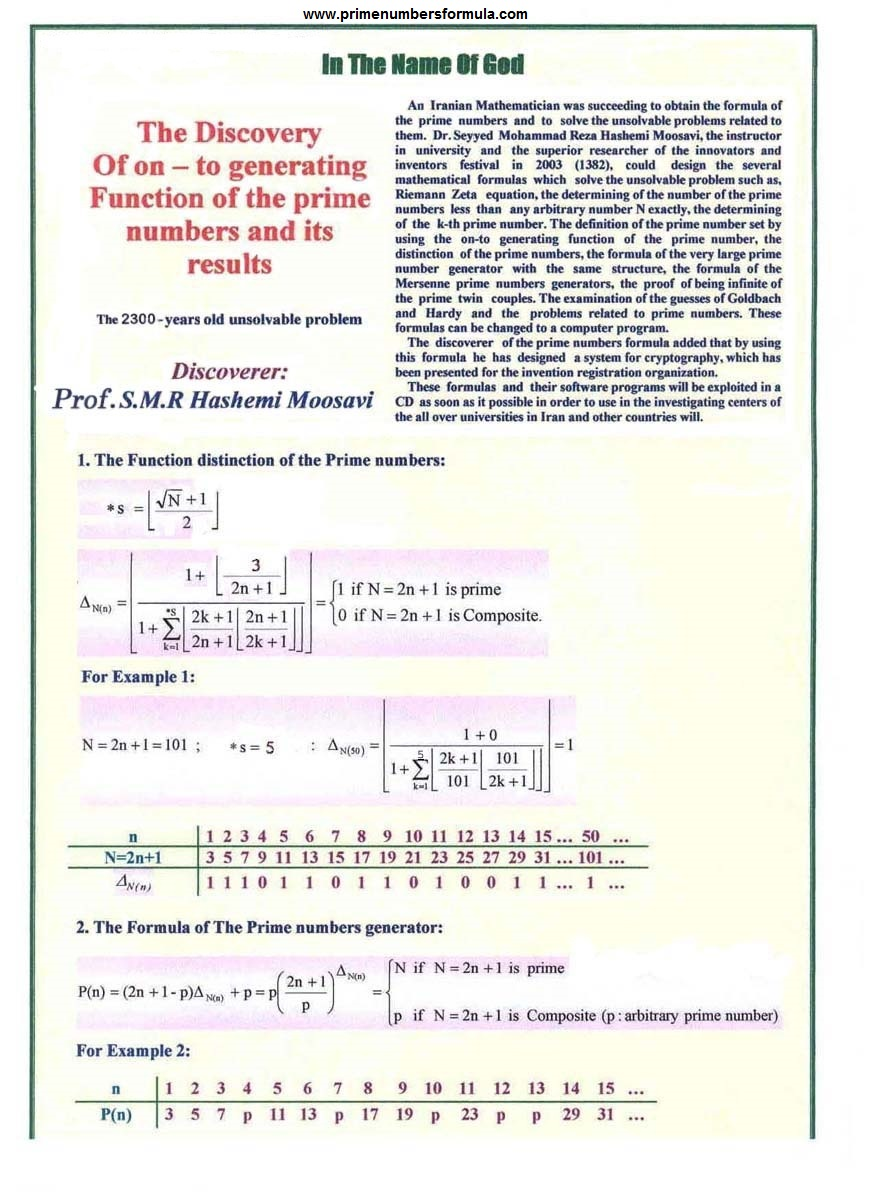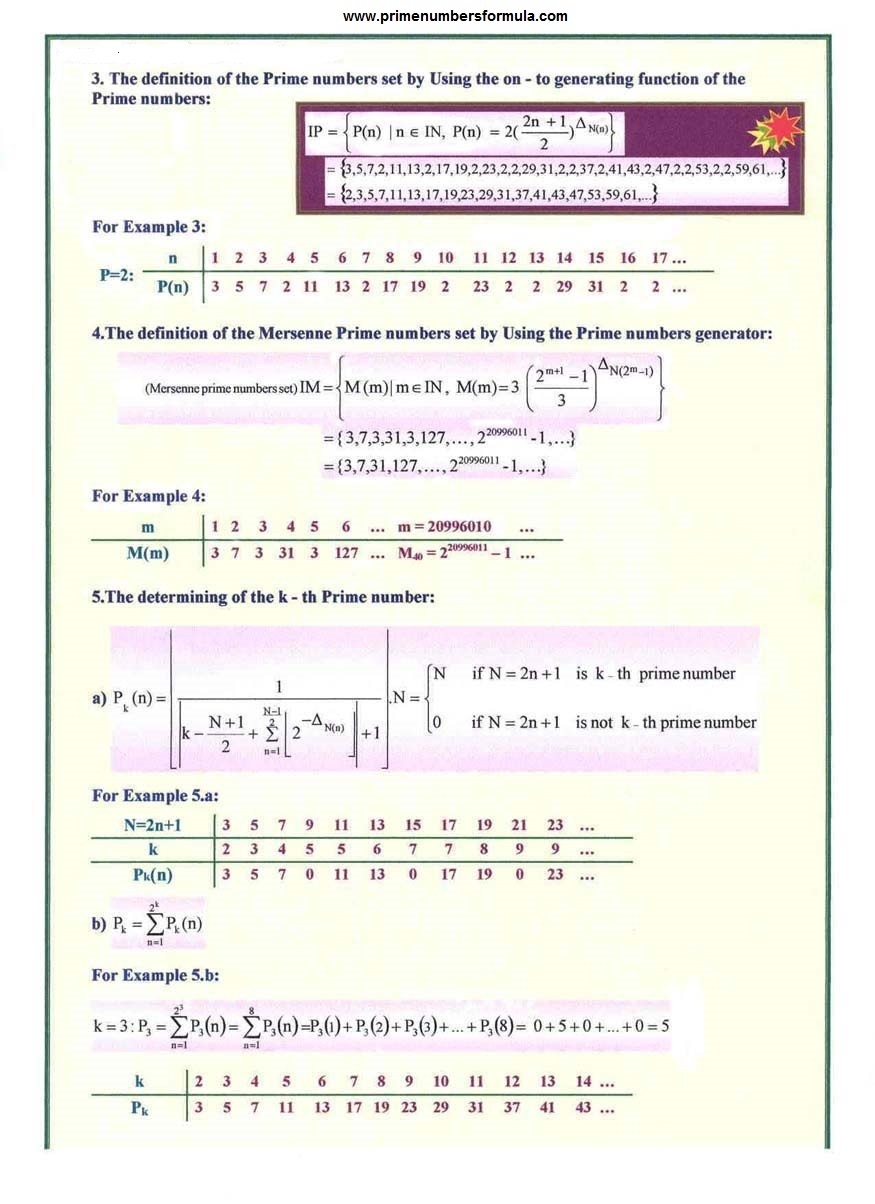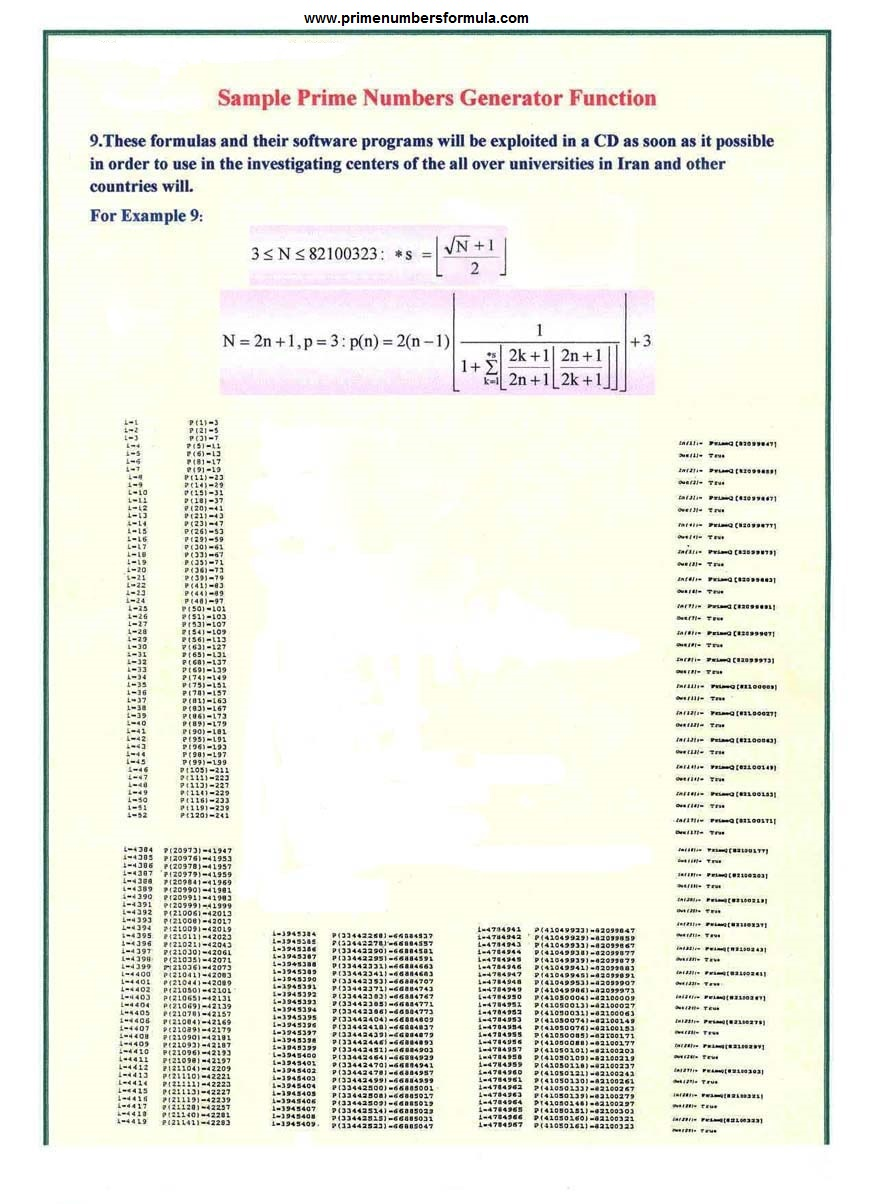You can freely download prime numbers formula software. Open source code of Seyyed Alireza Hashemi Moosavi's program is in follow that is based on H.M formula of prime numbers that was discovered in the year of 2003  Prof. Seyyed Mohammad Reza Hashemi Moosavi.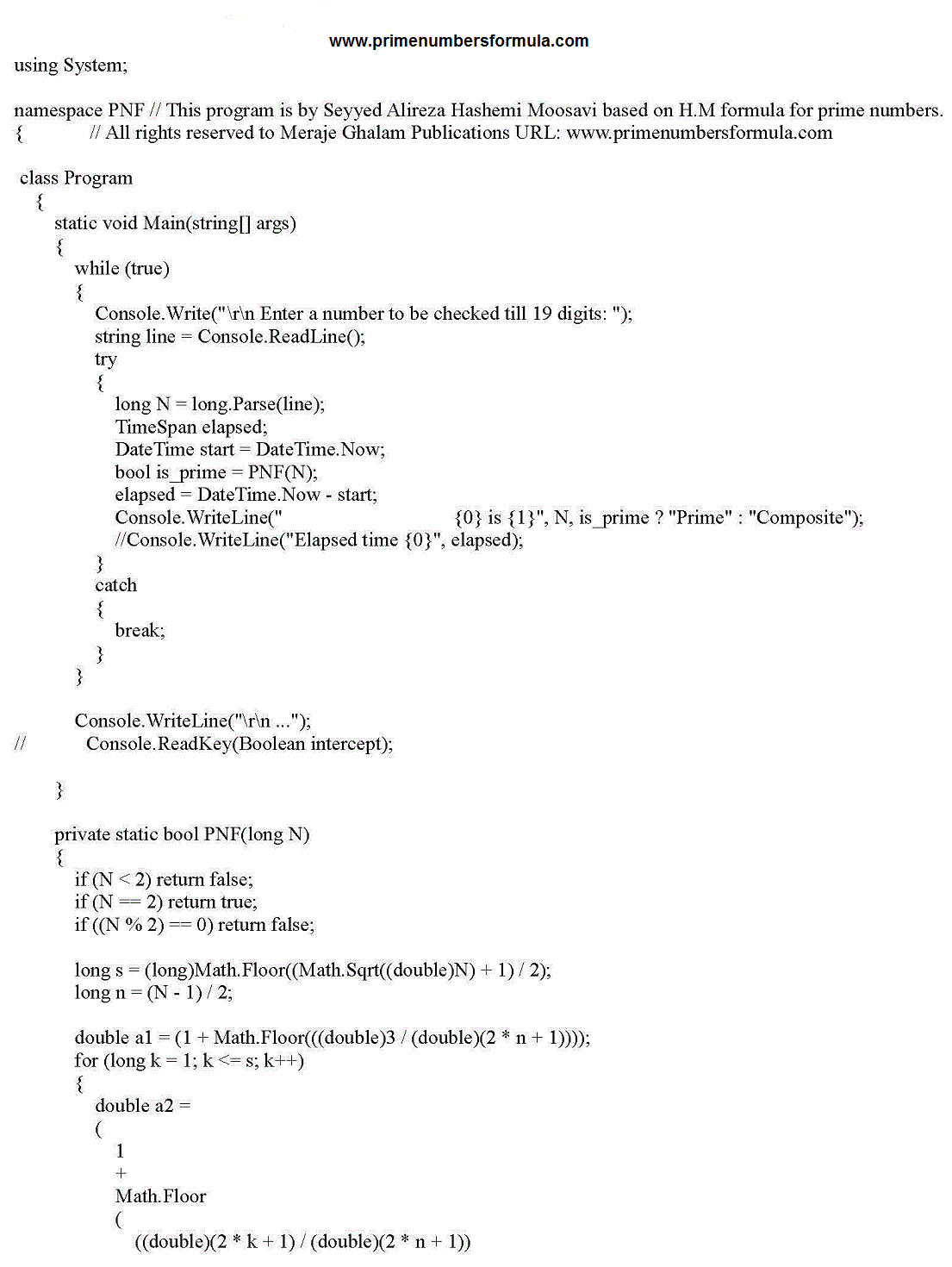### site : www.komhm.com

Take a look at new photo gallery

Note : This formula is one of the on-to generating functions for the prime   numbers that for every natural "m" it generates all the prime numbers (3,5,7,2,11,13,2,17,19, ...)

The presented "H.M"  functions by Discoverer (Prof. Seyyed Mohammad Reza Hashemi Moosavi) are 6 numbers that 4 functions are by Wilson's theorem and one of them by Euler's function(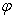) and one of them by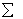functions and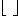functions are discovered by discoverer (Prof.S.M.R.Hashemi Moosavi). The software of this function is produced.Note :  In Year of 2007 (AAAS) " National Association Of Academies Of Science " (USA) Awarded an A++ = Excellent grade to prime numbers formula and its results by prof.S.M.R.Hashemi Moosavi.Note :  The discovery of prime numbers formula and its results has been published under an article in journal of "Roshd of Borhan " , associated with Ministry of Education.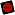More explain about discovery of prime numbers formula

Infinity proof of prime numbers was propound 300 years before the Christian era by Euclid and since that time great mathematician like Euler try to discover a formula for production of prime numbers.

Euler could define a quadratic function which give prime numbers for forty prime number which are uninterrupted and also Fermat presented a formula to obtain prime numbers and later, it breached by Euler for n = 5.

Many of other mathematicians achieved to violating and especially formula and finally they found that discovery of prime numbers formula is impossible and this problem will be unsolvable.

In fact this discovery means that one of complicated and unsolvable mathematics problem was solved and this discovery give this fact to man that earthy human can solve other unsolvable problems with research and effort.

I have worked about this problem around 20 years and I found this fact that I can't comeback from this path which I came and I promised that search about this un solvable problem till end of my life even if I couldn't achieve the final solution.

Of course my research had result with patronage under god and trust in god and I discovered the formula of prime numbers.How is the usage of this formula in mathematics  and other sciences?

A result for discovery of prime numbers formula is the solution of Riemann Zeta equation which is on of seven universal unsolvable problems in mathematics millennium and it's solution need to gain the number determination equation of prime number for any desirable number n carefully (with prime numbers formula).

Another result is determination of Kth desirable prime number and other usages are definition of prime number set, proof of infinity of prime twin pairs, considering of the guesses of Goldbuch and Hardy, gaining the generator formula of Mersenne prime numbers and also very unknown and big prime numbers and other problems related to prime numbers.

But the basic and cardinal usage of this formula is in coding and decoding that usually use from very big prime numbers for this and before it is necessary to gain them with complicated mathematics methods. But with presenting of this formula, definition of coding and decoding system became easy and I invent a system for coding with this formula that I presented this system inventions registration organization.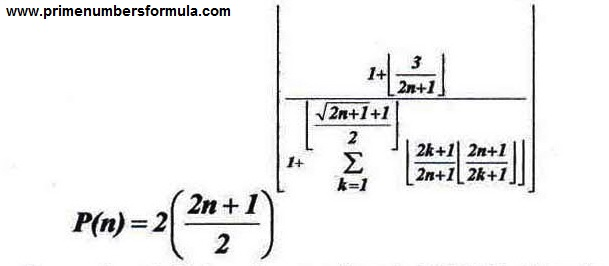After Euclid’s theory about infinite prime numbers in 300 B.C Most of the mathematicians and other researchers have been curious to find a formula which could generates prime numbers. After many years later, some mathematicians like Euler and Fermat presented some formulas to generate prime numbers limitedly.

Great mathematicians like Hardy and Courant and many other researchers officially announced that such a formula can’t be found and in follow to prove their wrong idea they started to publish some Algebraic theorems in their books.

Meanwhile, Niven and Mills in relation to prime numbers function proved the above theorem. But their parameters have never been determined.

Furthermore, determining the number of prime numbers was very important problem. So Gauss and other mathematicians started to set some tables for them.

We knew that there is no exact formula to determine the number of prime numbers exactly. This problem is known as Zeta Riemann equation which was one of the seven known unsolvable problems of the world that after my discovery on 5th August 2003, one of them is no more unsolvable with the prime numbers formula accurately you can absolutely generate all prime numbers to the nth one.

Its consequent generate of prime numbers formula resulted in defining the set of prime numbers and so many other unbelievable results until now like breaking the code of RSA and AES by the use of prime numbers formula and other sets like Mersenne prime, perfect numbers and so many important sets and results just related to the field of number theory and basic sciences.

• Discovery of prime numbers formula by Prof. Seyyed Mohammadreza Hashemi Moosavi caused so many results in basic sciences that we will mention a little part in follow:

• 1.         Distinction of prime numbers.

• 2.       Defining a formula for generating prime numbers.

• 3.       Definition of prime numbers set by using the generating function of prime numbers.

• 4.       Defining a formula to generate the Mersenne prime numbers.

• 5.       Determination of Nth prime number.

• 6.       Solving Riemann Zeta equation by using the determination of the number of prime number less than or equal to arbitrary number N exactly.

• 7.       The proof of guesses of Goldbuch and Hardy.

• 8.       The proof of infinity of the prime twin couples.

• 9.       Determining a general series of answer for Diophantine equations.

• 10.   This formula has so many unknown applications in Cryptography, generating Titan Mersenne prime numbers and other sciences like solving  NP.Click the link to download or open the PDF file of New Proof of Fermat's last Theorem

by Final - Main HM Theorem

Home | About Me | Article | Books | Photo Gallery | Contact

This site was last updated 11/23/20